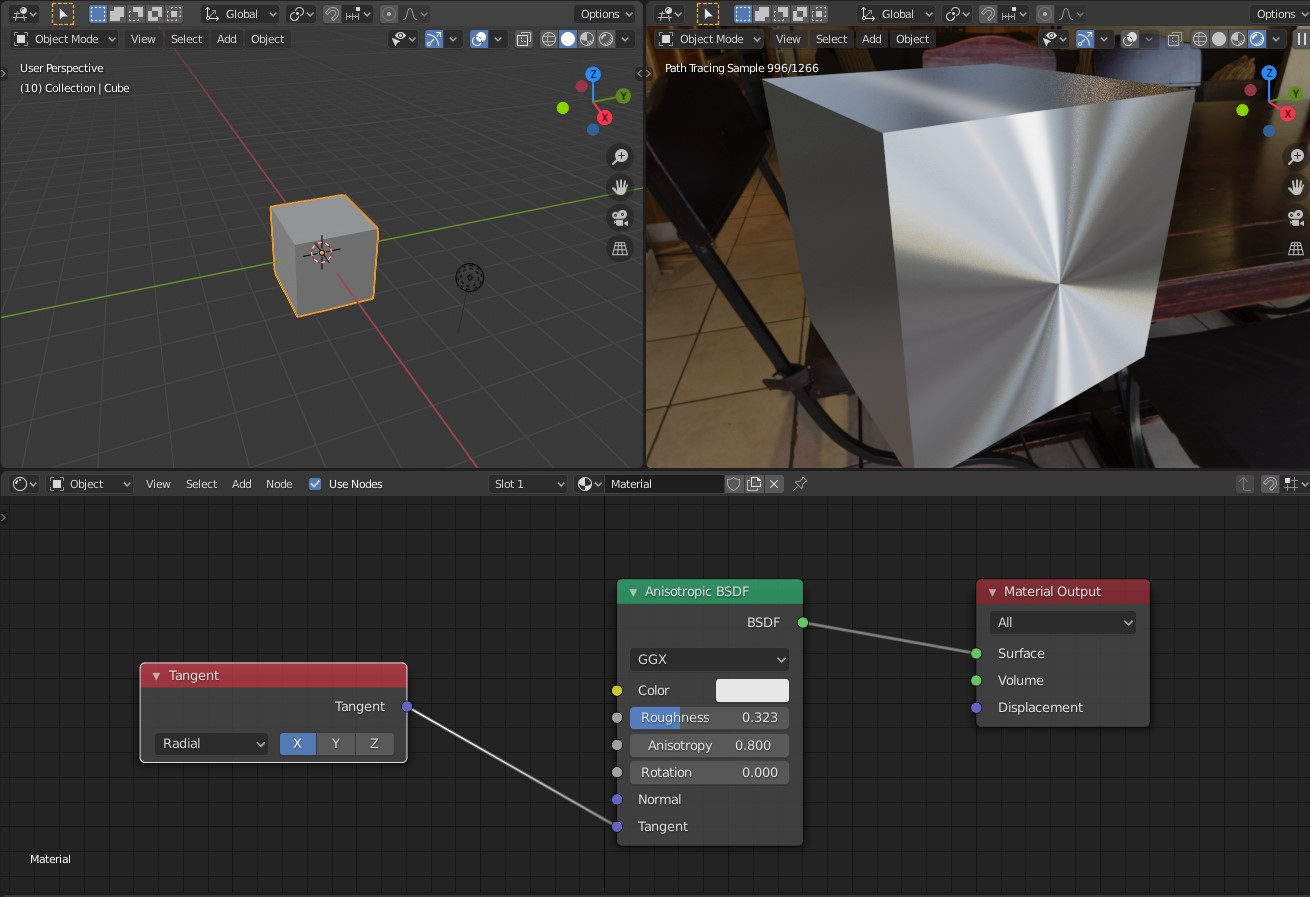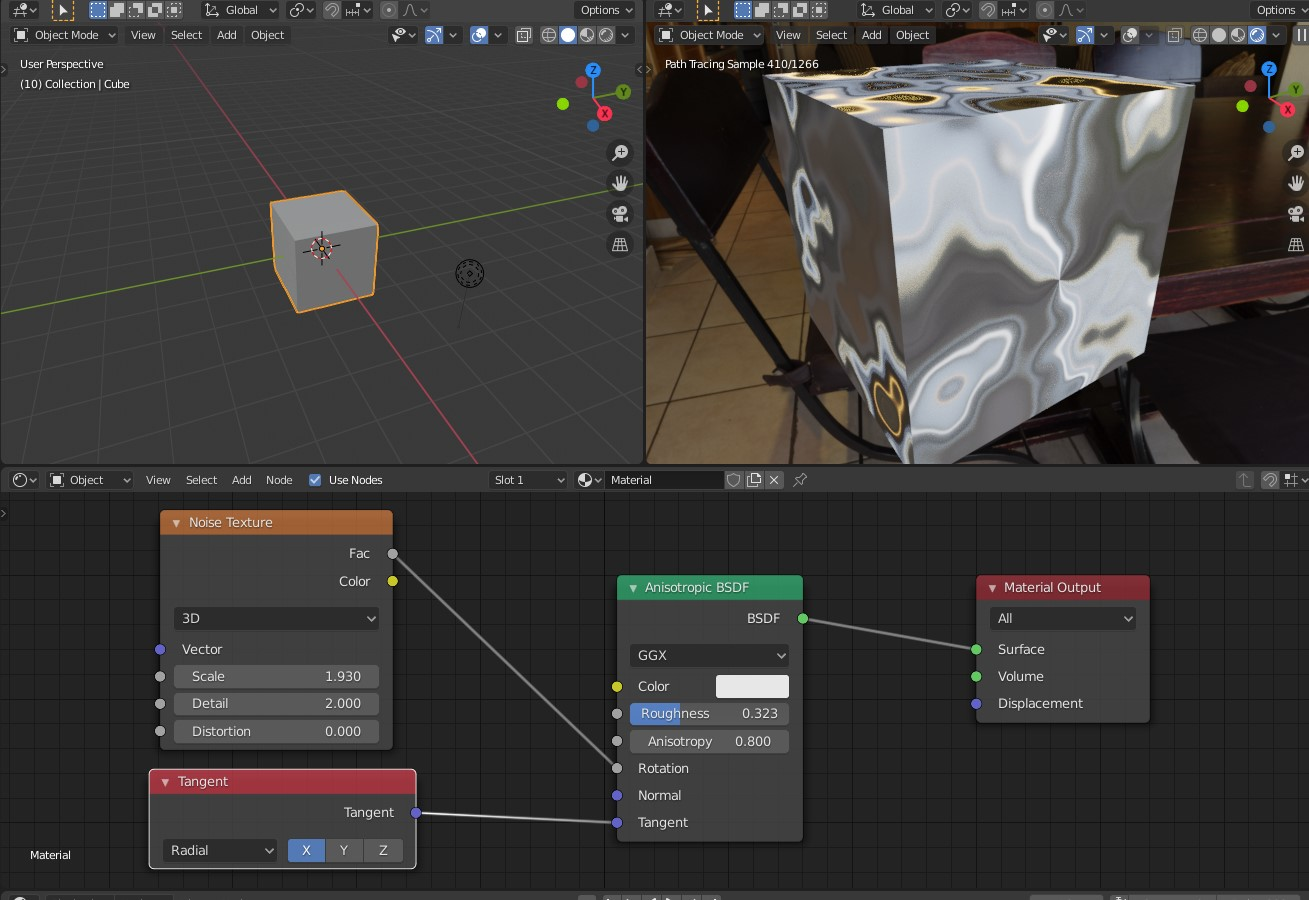## UE4 – Procedural 3D noise bump setups

Software:
Unreal Engine 4.25

Yet another case where I develop my own costly solution only to find out afterwards that there’s actually a much more efficient built-in solution.. 😀

In this case the subject is deriving a bump normal from a procedural or non-uv projected height map/texture (like noise, or tri-planar mapping for example).

The built-in way:
Using the pre-made material functions, PreparePerturbNormalHQ and PerturbNormalHQ, the first of which uses the low level Direct3D functions DDX and DDY to derive the two extra surface adjacent values needed to derive a bump normal, and the last uses the 3 values to generate a world-space bump normal:240 instructions
1. Noise coordinates are obtained by multiplying the surface shading point local position by a value to set the pattern density.
2. The Noise output value is multiplied by a factor to set the resulting bump intensity.
3. The PreparePerturbNormalHQ function is used to derive the 2 extra values needed to derive a bump normal.
4. The PerturbNormalHQ function is used to derive the World-Space bump normal.
5. Note:
Using this method, the material’s normal input must be set to world-space by unchecking Tangent Space Normal in the material properties.

The method I’m using:
This method is significantly more expensive in the number of shader instructions, but in my opinion, generates a better quality bump.
Sampling 3 Noise nodes at 3 adjacent locations in tangent-space to derive the 3 input values necessary for the NormalFromFunction material function:412 instructions
1. Noise coordinates are obtained by multiplying the surface shading point local position by a value to set the pattern density.
2. Crossing the vertex normal with the vertex tangent vectors to derive the bitangent (sometimes called “binormal”).
3. Multiplying the vertex-tangent and bitangent vectors by a bump-offset* factor to create the increment steps to the additional sampled Noise values.
* This factor should be parameter for easy tuning, since it determines the distance between the height samples in tangent space.
4. The increment vectors are added to the local-position to get the final height samples positions.
5. The NormalFromFunction material function is used to derive a tangent-space normal from the 3 supplied height samples.

Note:
From my experience, even though the UV1, UV2 and UV3 inputs of the NormalFromFunction are annotated as V3, the function will only work is the inputs are a scalar value and not a vector/color.

## Cycles Tangent node & Anisotropic reflection

Software:
Blender 2.82

The Cycles Tangent node defines a Tangent-Space for a mesh by either using one of the object’s local axes (see image A) or by using an available UV layout.
This can be useful when designing an Anisotropic reflection shader like in the case of brushed, machined or lathed metals.
A grey-scale texture can be connected to the Rotation input of the shader to define changes in the Anisotropic direction on the surface (see image B).

Examples:

A. In this example the Tangent node is set to ‘Radial’ Direction mode, in which a object axis is chosen as the radial axis of the Anisotropic direction:B. In this example a Noise texture’s Factor output is connected to the Anisotropic shader’s Rotation input to create an irregular Anisotropic (metal brushing finish) direction:Related: